# VBA Average – AVERAGE, AVERAGEA, AVERAGEIF

This tutorial will demonstrate how to use the Excel Average function in VBA.

The Excel AVERAGE Function is used to calculate an average from a range cells in your Worksheet that have values in them. In VBA, It is accessed using the WorksheetFunction method.

## AVERAGE WorksheetFunction

The WorksheetFunction object can be used to call most of the Excel functions that are available within the Insert Function dialog box in Excel. The AVERAGE function is one of them.

``````Sub TestFunction
Range("D33") = Application.WorksheetFunction.Average("D1:D32")
End Sub``````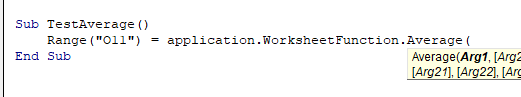You are able to have up to 30 arguments in the AVERAGE  function. Each of the arguments must refer to a range of cells.

This example below will produce the average of the sum of the cells B11 to N11

``````Sub TestAverage()
Range("O11") = Application.WorksheetFunction.Average(Range("B11:N11"))
End Sub``````

The example below will produce an average of the sum of the cells in B11 to N11 and the sum of the cells in B12:N12. If you do not type the Application object, it will be assumed.

``````Sub TestAverage()
Range("O11") = WorksheetFunction.Average(Range("B11:N11"),Range("B12:N12"))
End Sub``````

## Assign AVERAGE Result to a Variable

You may want to use the result of your formula elsewhere in code rather than writing it directly back to an Excel Range. If this is the case, you can assign the result to a variable to use later in your code.

``````Sub AssignAverage()
Dim result As Integer
'Assign the variable
result = WorksheetFunction.Average(Range("A10:N10"))
'Show the result
MsgBox "The average for the cells in this range is " & result
End Sub``````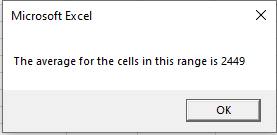## AVERAGE with a Range Object

You can assign a group of cells to the Range object, and then use that Range object with the WorksheetFunction object.

``````Sub TestAverageRange()
Dim rng As Range
'assign the range of cells
Set rng = Range("G2:G7")
'use the range in the  formula
Range("G8") = WorksheetFunction.Average(rng)
'release the range object
Set rng = Nothing
End Sub``````

## AVERAGE Multiple Range Objects

Similarly, you can calculate the average of the cells from multiple Range Objects.

``````Sub TestAverageMultipleRanges()
Dim rngA As Range
Dim rngB as Range
'assign the range of cells
Set rngA = Range("D2:D10")
Set rngB = Range("E2:E10")
'use the range in the formula
Range("E11") = WorksheetFunction.Average(rngA, rngB)
'release the range object
Set rngA = Nothing
Set rngB = Nothing
End Sub
``````

## Using AVERAGEA

The AVERAGEA Function differs from the AVERAGE function in that it create an average from all the cells in a range, even if one of the cells has text in it – it replaces the text with a zero and includes that in calculating the average.   The AVERAGE function would ignore that cell and not factor it into the calculation.

``````Sub TestAverageA()
Range("B8) = Application.WorksheetFunction.AverageA(Range("A10:A11"))
End Sub``````

In the example below, the AVERAGE function returns a different value to the AVERAGEA function when the calculation is used on cells A10 to A11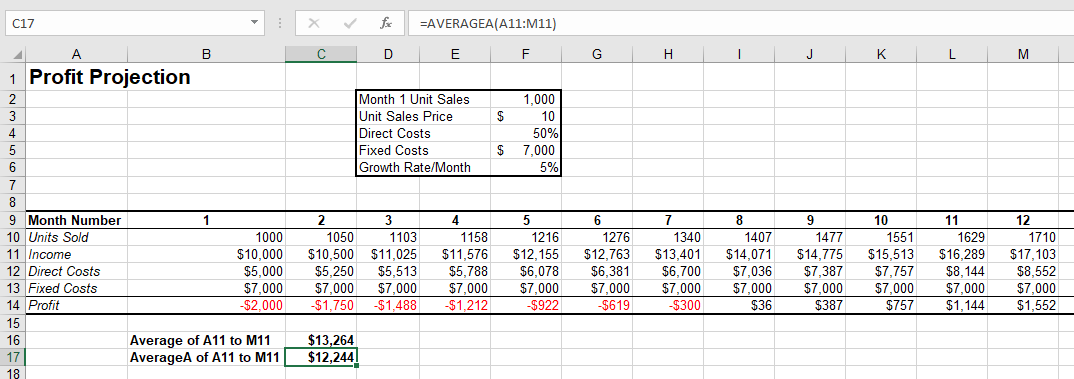The answer for the AVERAGEA formula is lower than the AVERAGE formula as it replaces the text in A11 with a zero, and therefore averages over 13 values rather than the 12 values that the AVERAGE is calculating over.

## Using AVERAGEIF

The AVERAGEIF Function allows you to average the sum of a range of cells that meet a certain criteria.

``````Sub AverageIf()
Range("F31") = WorksheetFunction.AverageIf(Range("F5:F30"), "Savings", Range("G5:G30"))
End Sub``````

The procedure above will only average the cells in range G5:G30 where the corresponding cell in column F has the word ‘Savings’ in it.  The criteria you use has to be in quotation marks.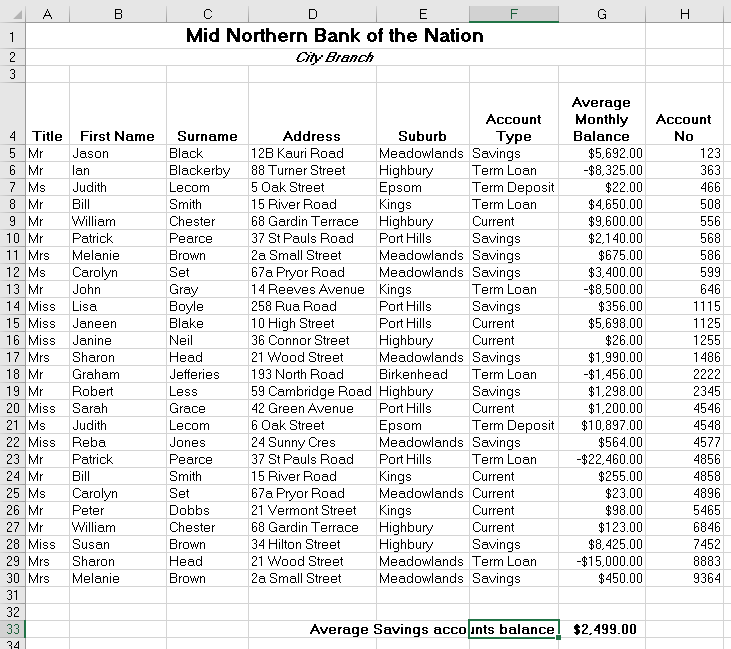Stop searching for VBA code online. Learn more about AutoMacro - A VBA Code Builder that allows beginners to code procedures from scratch with minimal coding knowledge and with many time-saving features for all users!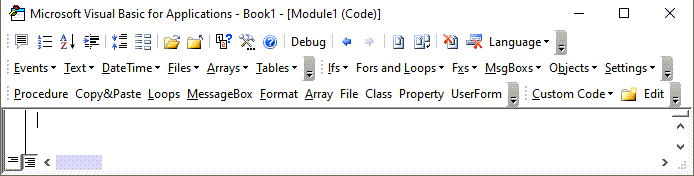When you use the WorksheetFunction to average the values in a range in your worksheet, a static value is returned, not a flexible formula. This means that when your figures in Excel change, the value that has been returned by the WorksheetFunction will not change.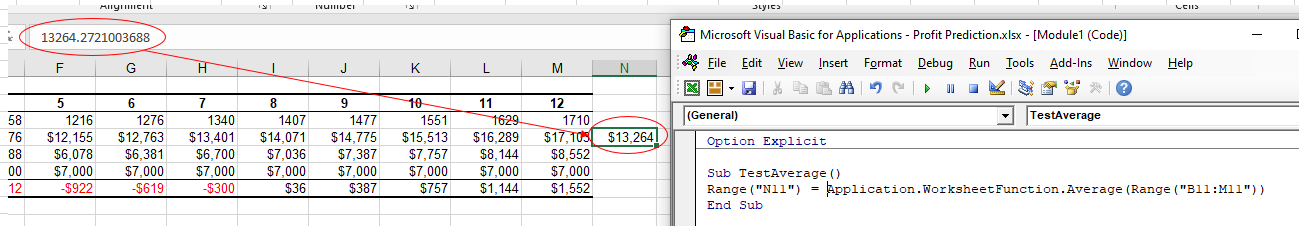In the example above, the procedure TestAverage procedure has created the average of B11:M11 and put the answer in N11.   As you can see in the formula bar, this result is a figure and not a formula.

If any of the values change therefore in the Range(B11:M11 ), the results in N11 will NOT change.

Instead of using the WorksheetFunction.Average, you can use VBA to apply the AVERAGE Function to a cell using the Formula or FormulaR1C1 methods.

### Using the Formula Method

The formula method allows you to point specifically to a range of cells eg: B11:M11 as shown below.

``````Sub TestAverageFormula()
Range("N11").Formula = "=Average(B11:M11)"
End Sub``````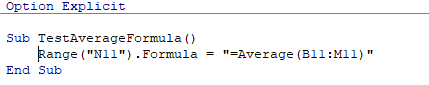### Using the FormulaR1C1 Method

The FomulaR1C1 method is more flexible in that it does not restrict you to a set range of cells. The example below will give us the same answer as the one above.

``````Sub TestAverageFormula()
Range("N11").Formula = "=Average(RC[-12]:RC[-1])"
End Sub``````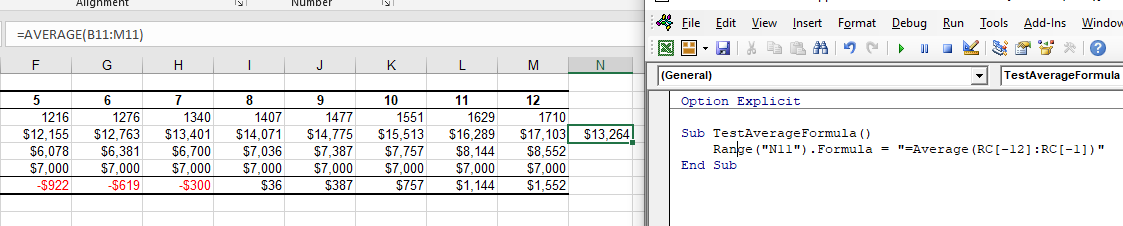However, to make the formula more flexible, we could amend the code to look like this:

``````Sub TestAverageFormula()
ActiveCell.FormulaR1C1 = "=Average(R[-11]C:R[-1]C)"
End Sub``````

Wherever you are in your worksheet, the formula will then average the values in the 12 cells directly to the left of it and place the answer into your ActiveCell. The Range inside the AVERAGE function has to be referred to using the Row (R) and Column (C) syntax.

Both these methods enable you to use Dynamic Excel formulas within VBA.

There will now be a formula in N11 instead of a value.